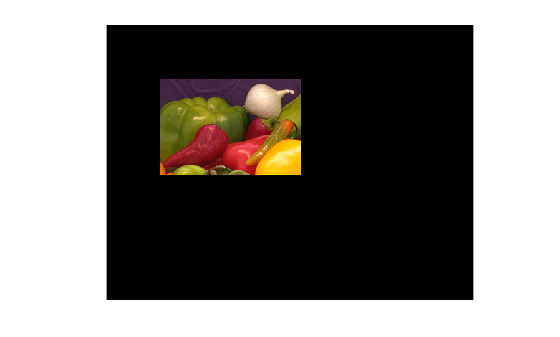# Registering an Image Using Normalized Cross-Correlation

This example shows how to find a template image within a larger image. Sometimes one image is a subset of another. Normalized cross-correlation can be used to determine how to register or align the images by translating one of them.

```onion = imread('onion.png'); peppers = imread('peppers.png'); imshow(onion)````figure, imshow(peppers)`### Step 2: Choose Subregions of Each Image

It is important to choose regions that are similar. The image `sub_onion` will be the template, and must be smaller than the image `sub_peppers`. You can get these sub regions using either the non-interactive script below or the interactive script.

```% non-interactively rect_onion = [111 33 65 58]; rect_peppers = [163 47 143 151]; sub_onion = imcrop(onion,rect_onion); sub_peppers = imcrop(peppers,rect_peppers); % OR % interactively %[sub_onion,rect_onion] = imcrop(onion); % choose the pepper below the onion %[sub_peppers,rect_peppers] = imcrop(peppers); % choose the whole onion % display sub images figure, imshow(sub_onion)````figure, imshow(sub_peppers)`### Step 3: Do Normalized Cross-Correlation and Find Coordinates of Peak

Calculate the normalized cross-correlation and display it as a surface plot. The peak of the cross-correlation matrix occurs where the sub_images are best correlated. `normxcorr2` only works on grayscale images, so we pass it the red plane of each sub image.

```c = normxcorr2(sub_onion(:,:,1),sub_peppers(:,:,1)); figure, surf(c), shading flat```### Step 4: Find the Total Offset Between the Images

The total offset or translation between images depends on the location of the peak in the cross-correlation matrix, and on the size and position of the sub images.

```% offset found by correlation [max_c, imax] = max(abs(c(:))); [ypeak, xpeak] = ind2sub(size(c),imax(1)); corr_offset = [(xpeak-size(sub_onion,2)) (ypeak-size(sub_onion,1))]; % relative offset of position of subimages rect_offset = [(rect_peppers(1)-rect_onion(1)) (rect_peppers(2)-rect_onion(2))]; % total offset offset = corr_offset + rect_offset; xoffset = offset(1); yoffset = offset(2);```

### Step 5: See if the Onion Image was Extracted from the Peppers Image

Figure out where `onion` falls inside of `peppers`.

```xbegin = round(xoffset+1); xend = round(xoffset+ size(onion,2)); ybegin = round(yoffset+1); yend = round(yoffset+size(onion,1)); % extract region from peppers and compare to onion extracted_onion = peppers(ybegin:yend,xbegin:xend,:); if isequal(onion,extracted_onion) disp('onion.png was extracted from peppers.png') end```
```onion.png was extracted from peppers.png ```

### Step 6: Pad the Onion Image to the Size of the Peppers Image

Pad the `onion` image to overlay on `peppers`, using the offset determined above.

```recovered_onion = uint8(zeros(size(peppers))); recovered_onion(ybegin:yend,xbegin:xend,:) = onion; figure, imshow(recovered_onion)```### Step 7: Use Alpha Blending To Show Images Together

Display one plane of the `peppers` image with the `recovered_onion` image using alpha blending.

`figure, imshowpair(peppers(:,:,1),recovered_onion,'blend')`